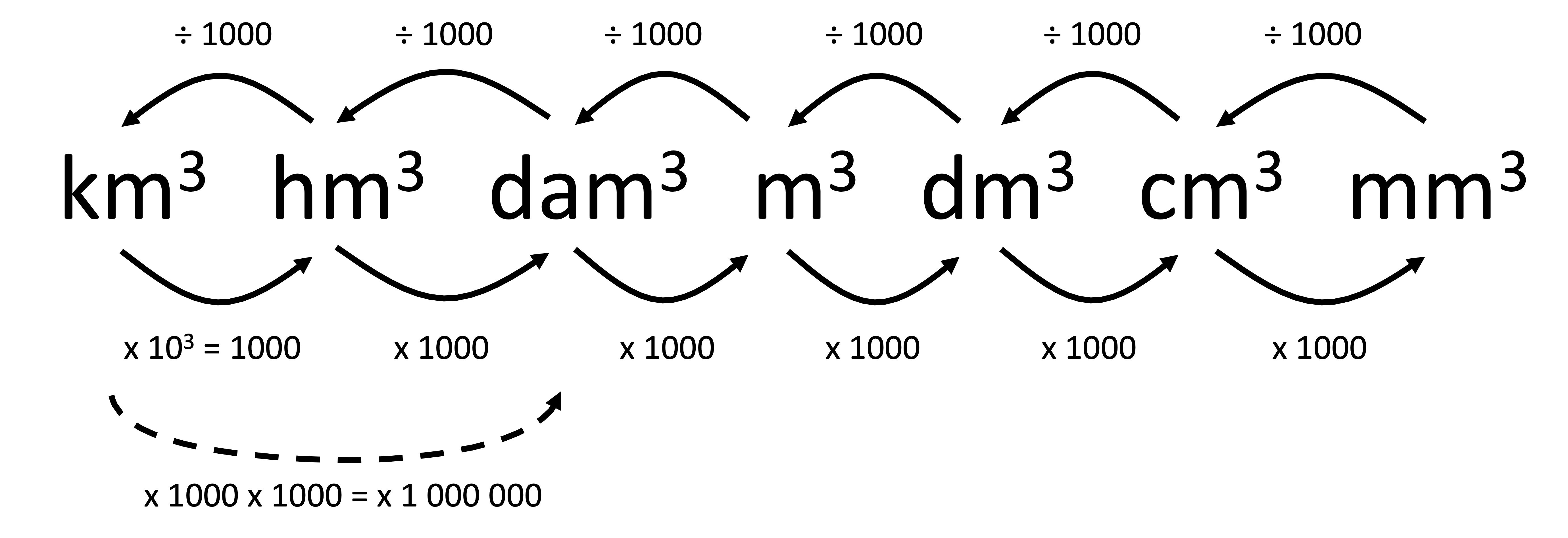# Help Zone

### Student Question

Hi,

How do you convert between units of volume (cubic meter, cubic millimeter, cubic decimeter, cubic centimeter, etc.)?

I do not understand!?!

Thank you

Science## Explanations (1)

•Explanation from AlloprofExplanation from Alloprof

This Explanation was submitted by a member of the Alloprof team.

To go from one unit of volume to another, you must know the conversion ratios from one unit to another:As you can see in the figure above, each passage from a larger unit of volume to a smaller unit of volume results in a multiplication by a factor of 1000. This corresponds to moving the decimal point three positions to the right. For example, to get from m^3 to dm^3, it is necessary to multiply by 1000.

Conversely, each change from a smaller unit of volume to a larger unit of volume generates a division by 1000. This corresponds to moving the decimal point three positions to the left. For example, to go from dam3 to hm3, you would have to divide by 1000.

When converting from more than one unit to another at the same time, you must multiply the conversion factors together.

For example, if converting m3 to cm3, one must multiply the given volume by 1000 • 1000 = 1,000,000, or move the decimal point by 6 positions to the right. Conversely, if one went from dm3 to hm3, they would have to divide by 1000 • 1000 • 1000 = 1,000,000,000, or move the decimal point by 9 positions to the left.

Don’t hesitate to ask other questions!